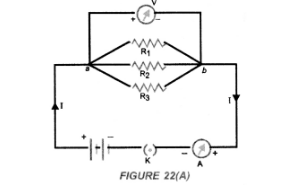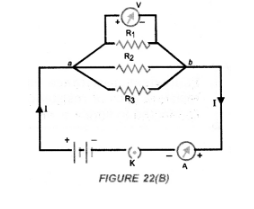# How will you conclude that the same potential

Question:

How will you conclude that the same potential difference (Voltage) exists across three resistors connected in parallel arrangement to a battery ?

Solution:

Perform an activity to investigate the relation between potential difference across parallel combination of resistors and the potential difference across each individual resistors,

1. Connect three resistors of resistances R1, R2 and R3 in parallel. One end of each resistor is joined at a common point ‘a’ and the other end of each resistor is connected at another common point ‘b’.
2. Connect the parallel combination of resistors with a battery, a plug key K and an ammeter A as shown in figure 22(A).3. Now connect a voltmeter across the parallel combination of resistors between a and b points.

4. Note the reading of voltmeter. Let it be $V$. This is the potential difference across the parallel combination of resistors.

5. Now, disconnect the voltmeter and connect it across $R_{1}$ as shown in figure $22(B)$.6. Note the reading of voltmeter. It is found to be $\mathrm{V}$.

7. Disconnect the voltmeter and connect it across $R_{2}$. Note the reading of voltmeter. It is found to be $V$.

8. Again disconnect the voltmeter and connect it across $R_{3}$. Note the reading of voltmeter. It is found to be $v$.

Conclusion : When resistors are connected in parallel to each other, potential difference across each resistor is equal to the potential difference across the parallel combination of resistors.